Next: Kepler's Third Law Up: Planetary Motion Previous: Kepler's Second Law

# Kepler's First Law

Our planet's radial equation of motion, (228), can be combined with Equation (247) to give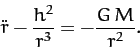(250)

Suppose that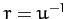. It follows that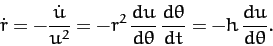(251)

Likewise,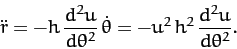(252)

Hence, Equation (250) can be written in the linear form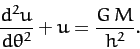(253)

The general solution to the above equation is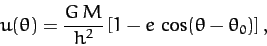(254)

where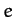and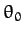are arbitrary constants. Without loss of generality, we can set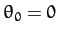by rotating our coordinate system about the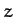-axis. Thus, we obtain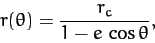(255)

where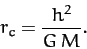(256)

We immediately recognize Equation (255) as the equation of a conic section which is confocal with the origin (i.e., with the Sun). Specifically, for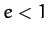, Equation (255) is the equation of an ellipse which is confocal with the Sun. Thus, the orbit of our planet around the Sun in a confocal ellipse--this is Kepler's first law of planetary motion. Of course, a planet cannot have a parabolic or a hyperbolic orbit, since such orbits are only appropriate to objects which are ultimately able to escape from the Sun's gravitational field.Next: Kepler's Third Law Up: Planetary Motion Previous: Kepler's Second Law
Richard Fitzpatrick 2011-03-31